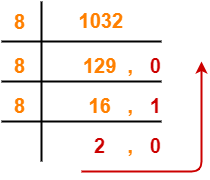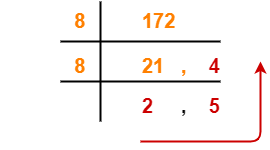## Number System Conversions-

Before you go through this article, make sure that you have gone through the previous article on Basics of Number System.

In number system,

• It is very important to have a good knowledge of how to convert numbers from one base to another base.
• Here, we will learn how to convert any given number from base 10 to base 8.## Decimal to Octal Conversion-

A given number can be converted from base 10 to any other base using division method and multiplication method.

Following two cases are possible-

### Case-01: For Numbers Carrying No Fractional Part-

• Division Method is used to convert such numbers from base 10 to another base.
• The division is performed with the required base.

### Steps To Convert From Base 10 to Base 8-

• Divide the given number (in base 10) with 8 until the result finally left is less than 8.
• Traverse the remainders from bottom to top to get the required number in base 8.

### Case-02: For Numbers Carrying A Fractional Part-

To convert such numbers from base 10 to another base, real part and fractional part are treated separately.

### For Real Part-

The steps involved in converting the real part from base 10 to another base are same as above.

### For Fractional Part-

• Multiplication Method is used to convert fractional part from base 10 to another base.
• The multiplication is performed with the required base.

### Steps To Convert From Base 10 To Base 8-

• Multiply the given fraction (in base 10) with 8.
• Write the real part and fractional part of the result so obtained separately.
• Multiply the fractional part with 8.
• Write the real part and fractional part of the result so obtained separately.
• Repeat this procedure until the fractional part remains 0.
• If fractional part does not terminate to 0, find the result up to as many places as required.

Required Number in Base 8

= Series of real part of multiplication results obtained in the above steps from top to bottom

## Problems-

Convert the following numbers from base 10 to base 8-

1. (1032)10
2. (1032.6875)10
3. (172)10
4. (172.878)10

## Solution-

### 1. (1032)10

(1032)10(?)8

Using division method, we have-From here, (1032)10 = (2010)8

### 2. (1032.6875)10

#### (1032.6875)10 → ( ? )8

Here, we treat the real part and fractional part separately-

### For Real Part-

• The real part is (1032)10
• We convert the real part from base 10 to base 8 using division method same as above.

So, (1032)10 = (2010)8

### For Fractional Part-

• The fractional part is (0.6875)10
• We convert the fractional part from base 10 to base 8 using multiplication method.

Using multiplication method, we have-

 Real part Fractional Part 0.6875 x 8 5 0.5 0.5 x 8 4 0.0

### Step-01:

• Multiply 0.6875 with 8. Result = 5.5.
• Write 5 in real part and 0.5 in fractional part.

### Step-02:

• Multiply 0.5 with 8. Result = 4.0.
• Write 4 in real part and 0.0 in fractional part.

Since fractional part becomes 0, so we stop.

• The fractional part terminates to 0 after 2 iterations.
• Traverse the real part column from top to bottom to obtain the required number in base 8.

From here, (0.6875)10 = (0.54)8

Combining the result of real and fractional parts, we have-

(1032.6875)10 = (2010.54)8

### 3. (172)10

#### (172)10 → ( ? )8

Using division method, we have-From here, (172)10 = (254)8

### 4. (172.878)10

#### (172.878)10 → ( ? )8

Here, we treat the real part and fractional part separately-

### For Real Part-

• The real part is (172)10
• We convert the real part from base 10 to base 8 using division method same as above.

So, (172)10 = (254)8

### For Fractional Part-

• The fractional part is (0.878)10
• We convert the fractional part from base 10 to base 8 using multiplication method.

Using multiplication method, we have-

 Real part Fractional Part 0.878 x 8 7 0.024 0.024 x 8 0 0.192 0.192 x 8 1 0.536 0.536 x 8 4 0.288

• The fractional part does not terminates to 0 after several iterations.
• So, let us find the value up to 4 decimal places.
• Traverse the real part column from top to bottom to obtain the required number in base 8.

From here, (0.878)10 = (0.7014)8

Combining the result of real and fractional parts, we have-

(172.878)10 = (254.7014)8

To gain better understanding about Decimal to Octal Conversion,

Watch this Video Lecture